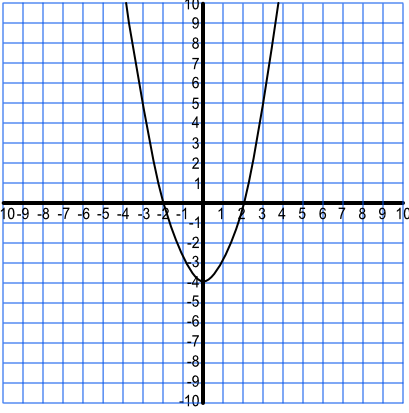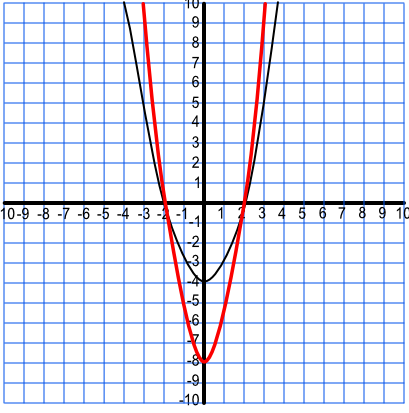Sketch Stretching of Functions

# Sketch Stretching of Functions

GCSE(H)

Graphs can be stretched horizontally and vertically by applying scale factors. If the factor is applied before the function is evaluated, then the stretching takes place parallel to the x-axis, but the amount of stretching is a reciprocal of the factor:

Mapping f(x) onto f(ax) stretches a graph by factor frac(1)(a) on the x-axis.

If the factor is applied after the function is evaluated, then the stretching takes place parallel to the y-axis:

Mapping f(x) onto af(x) stretches a graph by factor a on the y-axis.

## Examples

1. A graph has been transformed by a scale factor of 2. The point (4, 8) has been transformed to the point (2, 8). What scale factor has been applied to the function? Give your answer in terms of f(x).

Answer: f(2x)

The stretching has taken place along the x-axis, which means that the factor is being applied before the function is evaluated. The x value is half the original value, so the scale factor is frac(1)(2). Inverse the scale factor for f(2x).

2. A function y=f(x) is shown on the graph below. Sketch the graph for y=2f(x).Answer:The stretching is along the y-axis, by a factor of 2, as the factor is applied after the function has been evaluated.